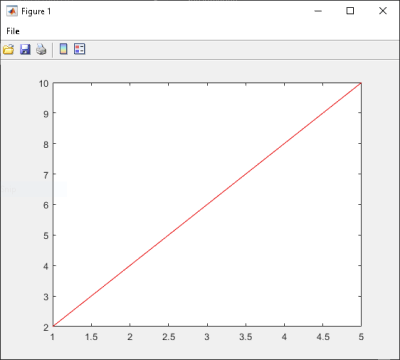## Integrate Function with Variable Number of Arguments

This example shows you how to create a .NET application using a MATLAB® function that takes a variable number of arguments instead of just one.

In this example, you perform the following steps:

• Use the MATLAB Compiler SDK™ product to convert the MATLAB function `drawgraph` to a method of a .NET class (`Plotter`) and wrap the class in a .NET assembly (`VarArgComp`). The `drawgraph` function displays a plot of the input parameters and is called as a method of the `Plotter` class.

• Access the component in a C# application (`VarArgApp.cs`) or a Visual Basic® application (`VarArgApp.vb`) by instantiating the `Plotter` class and using `MWArray` to represent data.

• Build and run the `VarArgDemoApp` application using the Visual Studio® .NET development environment.

### Files

 MATLAB Functions `drawgraph.m` `extractcoords.m` MATLAB Function Location `matlabroot\toolbox\dotnetbuilder\Examples\VSVersion\NET\VarArgExample\VarArgComp\` C# Code Location ```matlabroot\toolbox\dotnetbuilder\Examples\VSVersion\NET\VarArgExample\VarArgCSApp\VarArgApp.cs ``` Visual Basic Code Location `matlabroot\toolbox\dotnetbuilder\Examples\VSVersion\NET\VarArgExample\VarArgVBApp\VarArgApp.vb`

### Procedure

1. Copy the following folder that ships with the MATLAB product to your work folder:

```matlabroot\toolbox\dotnetbuilder\Examples\VSVersion\NET\VarArgExample ```

At the MATLAB command prompt, navigate to the new `VarArgExample\VarArgComp` subfolder in your work folder.

2. Examine the `drawgraph` and `extractcoords` functions.

```function [xyCoords] = DrawGraph(colorSpec, varargin) numVarArgIn= length(varargin); xyCoords= zeros(numVarArgIn, 2); for idx = 1:numVarArgIn xCoord = varargin{idx}(1); yCoord = varargin{idx}(2); x(idx) = xCoord; y(idx) = yCoord; xyCoords(idx,1) = xCoord; xyCoords(idx,2) = yCoord; end xmin = min(0, min(x)); ymin = min(0, min(y)); axis([xmin fix(max(x))+3 ymin fix(max(y))+3]) plot(x, y, 'color', colorSpec); ```
```function [varargout] = ExtractCoords(coords) for idx = 1:nargout varargout{idx}= coords(idx,:); end ```

3. Build the .NET component with the Library Compiler app or `compiler.build.dotNETAssembly` using the following information:

FieldValue
Library Name`VarArgComp`
Class Name`Plotter`
Files to Compile`extractcoords.m`   `drawgraph.m`

For example, if you are using `compiler.build.dotNETAssembly`, type:

```buildResults = compiler.build.dotNETAssembly(["extractcoords.m","drawgraph.m"], ... 'AssemblyName','VarArgComp', ... 'ClassName','Plotter');```

For more details, see the instructions in Generate .NET Assembly and Build .NET Application.

4. Decide whether you are using C# or Visual Basic to access the component.

• C#

If you are using C#, write source code for a C# application that accesses the component.

The sample application for this example is in `VarArgExample\VarArgCSApp\VarArgApp.cs`.

The following statements are alternative ways to call the `drawgraph` method:

``````data= (MWNumericArray)plotter.drawgraph(colorSpec, coords, coords, coords,coords, coords); ... data= (MWNumericArray)plotter.drawgraph((MWArray)colorSpec, coords); ``````
• Visual Basic

If you are using Visual Basic, write source code for a Visual Basic application that accesses the component.

The sample application for this example is in `VarArgExample\VarArgVBApp\VarArgApp.vb`.

The following statements are alternative ways to call the `drawgraph` method:

``````data = CType(plotter.drawgraph(colorSpec, coords(0), coords(1), coords(2), coords(3), coords(4)), MWNumericArray) ... data = CType(plotter.drawgraph(colorSpec, coords), MWNumericArray)``````

In either case, the `VarArgApp` program does the following:

• Initializes three arrays (`colorSpec`, `data`, and `coords`) using the `MWArray` class library

• Creates a `Plotter` object

• Calls the `extracoords` and `drawgraph` methods

• Uses `MWNumericArray` to represent the data needed by the methods

• Uses a `try-catch` block to catch and handle any exceptions

5. Open the .NET project file that corresponds to your application language using Visual Studio.

• C#

If you are using C#, the `VarArgCSApp` folder contains a Visual Studio .NET project file for this example. Open the project in Visual Studio .NET by double-clicking `VarArgCSApp.csproj` in Windows® Explorer. You can also open it from the desktop by right-clicking VarArgCSApp.csproj and selecting Open Outside MATLAB.

• Visual Basic

If you are using Visual Basic, the `VarArgVBApp` folder contains a Visual Studio .NET project file for this example. Open the project in Visual Studio .NET by double-clicking `VarArgVBApp.vbproj` in Windows Explorer. You can also open it from the desktop by right-clicking VarArgVBApp.vbproj and selecting Open Outside MATLAB.

6. Create a reference to your assembly file `VarArgComp.dll` located in the folder where you generated or installed the assembly.

7. Create a reference to the `MWArray` API.

 If MATLAB is installed on your system `matlabroot\toolbox\dotnetbuilder\bin\win64\\MWArray.dll` If MATLAB Runtime is installed on your system `\toolbox\dotnetbuilder\bin\win64\\MWArray.dll`

8. Build and run the `VarArgApp` application in Visual Studio .NET.

The program displays the following output:

```result= 1 2 2 4 3 6 4 8 5 10 ```